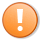# Tutorial 109 | Plotting the duration of volume bars in seconds

## How to calculate the time span of volume bars

A new tutorial describing a real-time version of this tutorial is available here.

A markplex.com customer asked “how to calculate and plot the duration of bars on a volume bar chart.” Tutorial 109 is a TradeStation EasyLanguage programming tutorial that demonstrates how to create an indicator to calculate the length of time of a volume bar.

This simple program is available free to Gold Pass members (see below), others may type in the program or download it for \$4.95.

The following video explains the development of the indicator:

### Programming issues

Tutorial 109 is a fairly easy program to understand, however, there are a couple of areas that may need further explanation.

In the very basic version of the program we calculate the duration of the bar by subtracting the time of the last tick of the previous bar from the time of the closing tick of the volume bar for which we are trying to find the duration. This assumes that the closing time of the last bar is the same as the opening time of the bar we are looking at. This is not the case. For example, when a new day begins on the @ES.D chart, the closing time of the last bar of the previous day took place some considerable time before the opening of the first bar of the new day.

To overcome this issue I tested to see if the bar was the first of a new day and if so, I calculated its length based on the session start time. This works for @ES.D and may be an approximation but is probably close enough.

When real time bars start to arrive we are now able to record the start time and end time of each bar.

Tutorial 109 demonstrates the TimeSpan and DateTime objects that make it easy to manipulate date and time information to the second.

### Gold pass areaThis content is for members only.